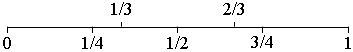SEARCH HOMEMath Central Quandaries & QueriesQuestion from Myon, a student: My problem is putting fractions from least to greatest and they are 1/4 2/5 1/3 4/6 1/2 8/9 3/4 1/1 2/6 1/10 thanksMyon,

First you should put each of the fractions in lowest terms.

If you go to the Quandaries and Queries page on Math Central and use the Quick Search to search for the term ordering fractions you will find a number of responses to similar questions that will help with this.

For this problem I would draw the interval from 0 to 1 and place common fractions on it, $\frac12, \frac14, \frac34, \mbox{ as well as }\frac13 \mbox{ and } \frac23.$Which of your fractions are included in the diagram?

For the remaining fractions, one at a time decide where you think they should go and use the common denominator technique described in our responses to similar questions to determine if you are correct.

PennyMath Central is supported by the University of Regina and The Pacific Institute for the Mathematical Sciences.